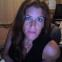# Do Now Lesson 64

 Name:

1. The only "natural" motion is for an object to be at rest.
 True False

1. If an object is at rest, no forces are acting on the object.
 True False

1. An object has force and when it runs out of force it stops moving.
 True False

1. A force is needed to keep an object moving with a constant speed.
 True False

1. The acceleration of an object is always in the direction of the net force applied to the object.
 True False

1. If two objects are both at rest, they have the same amount of inertia.
 True False

1. The product of mass and acceleration, m*a, is a force.
 True False

1. The normal force on an object always equals the weight of the object.
 True False

1. Equilibrium means that all the forces on an object are equal.
 True False

1. A force applied by, say a hand, only acts on an object until the object leaves the hand.
 True FalseMrs. Burns Pascack Hills High School Montvale, NJ